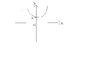# Multivariable calculus question

• engineer_dave

## Homework Statement

Sketch the following curves on separate number planes.

y= 2x^2

x^2 + 2y^2= 4

## The Attempt at a Solution

For the first one, would the intersection of the curve with the y-axis be at y=2 ( I have attached a diagram of my solution). The minimum point would be at (0,2)?

For the second one, what do we do with the 2 which is the coefficient of y^2. Would the 4 be the radius of the circle.

#### Attachments

•Curve.JPG
3.7 KB · Views: 412

In the first one. If the curve was intersecting the y-axis, then x = 0 at the point of intersection, and so y = 0 aswell, so it intersects are (0,0). Also, the minimum point of the function is at (0,0).

For the second one, are you sure that the equation you have describes a circle? I would call it an ellipse myself.

oh alrite, but then what happens with the 2 in x^2 for the first equation. Yea, the second one is an ellipse so would 4 be the radius?? Cheers

$$y = 2x^2$$
if x = 0; $$y = 2*(0^2) = 0$$

As for the ellipse. have you tried working out the values for x and y when the other one is set to 0? That will tell u where it crosses each axis.

edit: This might help - http://en.wikipedia.org/wiki/Ellipse

Last edited: